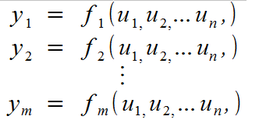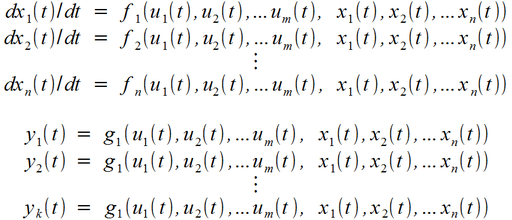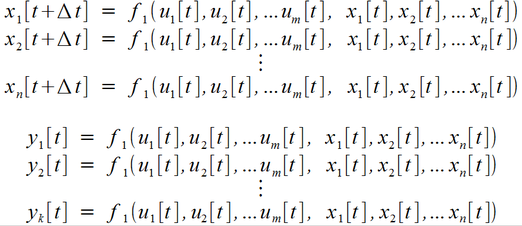# Simulation - Static vs. Dynamic Models - EdsCave

Go to content

## Simulation - Static vs. Dynamic Models

Simulation > Introduction to Simulation

6 July 2017

One major way of classifying simulation models is whether they are static or dynamic.

A static model is one which contains no internal history of either input values previously applied, values of internal variables, or output values. A canonical example of a static model is  a set of algebraic equations.In this type of model, each output (y i ) is dependent on some function (f i ) of the inputs (u i ). It is also possible for the output variables to be dependent on other output variables, although this results in a model which may not have closed-form (simple) solutions.   Functions are not necessarily limited to algebraic expressions – look-up and interpolation tables, as well as non-numeric operations can also be used in static models.

To 'run' this type of static simulation model involves setting the parameters of the equations, providing values for any inputs they may require, and evaluating, which yields a set of results.  A static model can be thought of as providing a 'snapshot' of a system's response to a specified set of input conditions.

An example of a static model is the calculation of mechanical stress in a bridge or other similar structure. Although in actual use, cars and trucks may be driven over it, one of the most basic models that will typically be built is one which assumes that some weight distribution has been placed on the bridge at some point in the past, and that everything has settled into a steady-state condition. While this type of model does not account for the effects of moving traffic, wind, earthquakes, or other things that vary in time, it does provide a very useful first-order analysis of whether the bridge can support the weight it is required to.  If the stresses in any of the statically simulated bridge's structural components exceeds (or even approaches) their rated failure  limits, it is time to go back to the drawing board to modify the design.

Since a static simulation model of a bridge won’t account for the effects of loads created by time-varying phenomena such as moving traffic, wind and earthquakes,  there are a lot of interesting behaviors it can't model – namely those effects which manifest themselves only though time.   Dynamic models provide one means of simulating the time-dependent behavior of systems.

The defining feature of a dynamic model is that unlike the static model, it does maintain an internal 'memory' of some combination of prior inputs, internal variables, and outputs.  The canonical example of a dynamic model involves the combination of algebraic and differential equations:In the above model, there are still input variables (u i ) and output variables (y i ). The main addition is a series of state variables (x i ) that are dependent on both the input variables and themselves.  The output variables (y i (t)) are defined by a set of functions 'g' whose outputs are also dependent on the present state of the input variables and the state variables.

Also note that all variables are now denoted as functions of time.  While you can certainly formulate a static model such that the inputs and outputs are functions of time, it is much more so important to specify this in a dynamic model.  In the static model,  providing the same set of input values will always result in the same set of output values.  In the case of the dynamic model,  the output values at any instant in time are dependent on not only the input values at that time, but may also be dependent on all of the input values presented to the model at previous times as well.

As previously mentioned, a dynamic model has internal memory – this is provided by the state variables.    The values of the state variables in this example are  not defined, just their rate of change (dx/dt), which can (and for most interesting models, will) vary as a function of time.  And that rate of change is dependent on their current values, and the current values of the inputs.  You may recognize the state variables and their defining  relationships as an example of  set of 1 st  order differential equations.

Since only the rate of change is defined for the state variables,  consider what happens if for some interval of time, that rate of change were somehow to be zero – the values of the state variables would just remain constant from the start to the end of that time interval. Even in the more common case where their rate of change is not zero, but is some other value, their ability to change is limited by the defining rate of change. This means that their values tend to change only a small amount when viewed from instant-to-instant, and this is the essence of how state variables provide a system with memory.

Another consequence of defining only the rate of change for the state variables is that we must also define initial conditions for them -  values at t=0, or whatever other point we wish to begin the simulation from.  And that leads to another characteristic of a dynamic simulation model – time is the independent variable that drives everything else.  Input variables are defined either as functions of time or as constants (which can still be thought of as  very dull functions of time whose values do  not change!).  Time is given a privileged status within the model and is often referred to as the simulation clock.  In implementations of simulations, the time variable is often managed implicitly  by the software, and cannot be directly controlled by conditions in the model. For example, in a popular electronic circuit simulation program called SPICE, the user will specify a stop-time for the simulation (the simulation assumes the start-time is t=0), and a time increment to use for periodically reporting the simulated circuit's status. The actual  progression of the 'time' variable within the simulation model is handled automatically, and mostly invisibly within the simulation software.

Differential equations are most useful in describing systems where the state variables change continuously, that is, the values of the state variables do not change in discrete jumps.  Because many physical systems behave this way, and many other systems' behavior can be approximated by continuous change, the differential equation paradigm is extremely useful in a wide range of modeling domains.

Some systems really are not modeled very well by continuously changing variables. In physics, quantum mechanics is all about the idea that at the most fundamental levels of reality, the world becomes discontinuous.   Outside of this specialized field of physics, however, the most common models in which discontinuous behavior occurs may be found in man-made systems.  One familiar example is a traffic signal. In the U.S., when changing from a 'go' to a 'stop' signal the signaling device will sequence green, yellow and red lamps in that order. There may be a brief instant where two lamps are illuminated, but this is ignored for practical purposes, and claiming the light was 'orange' is when you ran it is unlikely to get your ticket dismissed in court. Another example of a discontinuous system is the price of a  stock in a financial market. Although when plotted on a chart it may seem if the price varies continuously, more detailed observation will show that it really changes in discrete steps (often \$0.01) at discrete times.

While it is often possible to model discontinuous systems using the continuous differential equation paradigm, there are other representations which are often better suited for this purpose – better suited as in requiring fewer approximations and intellectual somersaults to form a useful model. A state machine is one such model which is intended specifically for representing highly discontinuous systems.  One formulation of a state machine is shown in the set of equations below:Similarly to the continuous model,  the discrete model has input variables (ui[t]), output variables (yi[t]) and state variables (xi[t]). The key differences from the continuous differential-equation model are:

• Time in the simulation progresses in discrete steps of 'Δt'.  Nothing interesting happens between these discrete time-points.

• The variables do not represent continuous functions – they  only need to be defined for specific points in time, and are represented as discrete sequences of numbers.   While their values may often be treated as persisting between the time steps, this is not necessary to 'executing' the simulation.

• State variables are updated with completely new values (x[t+ Δt]) based on their previous values (x[t]) and the previous values of the inputs (u[t]).  Unlike the state variables in the differential equation paradigm, the state variables in a state machine paradigm can, and always do, change in discrete steps.

The above set of equations should be treated as the 'entry-level' paradigm for modeling discontinuous or discrete systems. There are many variations possible on the basic scheme described above, which are often chosen to simplify the modeling task for a particular system or class of systems.  One common example is that the  Δt time-steps in a simulation do not necessarily have to be uniform. Some systems do not experience state updates on a regular schedule, and requiring a uniform time step may require that the whole model be re-evaluated  very frequently, so not as to 'miss' any important events. Allowing the model to dynamically vary the time step can be very useful in these situations, as the time step can be made smaller during intervals where more where the modeled system has more activity (and you don't want to inadvertently skip over significant events), and larger at times when there is less activity (and repeatedly evaluating the system would be computationally wasteful).

It is also possible for a dynamic model to include both continuous and discontinuous components. In this case the model will include both differential equations and state machines.  While this does make the low-level implementation of the simulation (in software) significantly more complex, it also can result in significantly improved modeling capability – allowing for both more natural modeling of complex systems, and higher-fidelity simulation results.